home | O'Reilly's CD bookshelfs | FreeBSD | Linux | Cisco | Cisco Exam

#Chapter 12nroff and troff## 12.9 Special Characters

This section lists the following special characters:

• Characters that reside on the standard fonts

• Miscellaneous characters

• Bracket-building symbols

• Mathematics symbols

• Greek characters

The characters in the first table below are available on the standard fonts. The characters in the remaining tables are available only on the special font.

Table 12.2: Characters on the Standard Fonts
Input Char Character Name
```' ``` ' Close quote
```` ``` ` Open quote
```\(em ```  -- Em-dash (width of "m")
```\(en ``` - En-dash (width of "n")
```\- ``` - Minus in current font
```- ``` - Hyphen
```\(hy ``` - Hyphen
```\(bu ```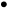Bullet
```\(sq ```Square
```\(ru ``` _ Rule
```\(14 ``` ¼ 1/4
```\(12 ``` ½ 1/2
```\(34 ``` ¾ 3/4
```\(fi ```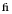fi ligature
```\(fl ```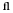fl ligature
```\(ff ```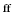ff ligature
```\(Fi ```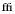ffi ligature
```\(Fl ```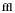ffl ligature
```\(de ``` ° Degree
```\(dg ```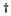Dagger
```\(fm ``` ' Foot mark
```\(ct ``` ¢ Cent sign
```\(rg ``` ® Registered
```\(co ``` © Copyright

Table 12.3: Miscellaneous Characters
Input Char Character Name
```\(sc ``` § Section
```\(aa ``` ´ Acute accent
```\´ ``` ´ Acute accent
```\(ga ``` ` Grave accent
```\` ``` ` Grave accent
```\(ul ``` _ Underrule
```\(-> ```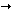Right arrow
```\(<- ```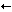Left arrow
```\(ua ```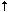Up arrow
```\(da ```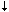Down arrow
```\(br ``` | Box rule
```\(dd ```Double dagger
```\(rh ```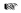Right hand
```\(lh ```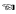Left hand
```\(ci ```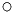Circle

Table 12.4: Bracket-Building Symbols
Input Char Character Name
```\(lt ```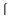Left top of big curly bracket
```\(lk ```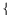Left center of big curly bracket
```\(lb ```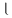Left bottom of big curly bracket
```\(rt ```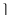Right top of big curly bracket
```\(rk ```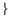Right center of big curly bracket
```\(rb ```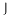Right bottom of big curly bracket
```\(lc ```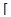Left ceiling (left top) of big square bracket
```\(bv ``` | Bold vertical
```\(lf ```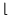Left floor (left bottom) of big square bracket
```\(rc ```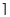Right ceiling (right top) of big square bracket
```\(rf ```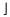Right floor (right bottom) of big square bracket

Table 12.5: Mathematics Symbols
Input Char Character Name
```\(pl ``` + Math plus
```\(mi ``` - Math minus
```\(eq ``` = Math equals
```\(** ``` * Math star
```\(sl ``` / Slash (matching backslash)
```\(sr ```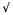Square root
```\(rn ``` - Root en extender
```\(>= ```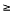Greater than or equal to
```\(<= ```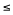Less than or equal to
```\(== ```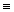Identically equal
```\(~~ ```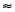Approximately equal
```\(ap ``` ~ Approximates
```\(!= ```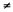Not equal
```\(mu ``` × Multiply
```\(di ``` ÷ Divide
```\(+- ``` ± Plus-minus
```\(cu ```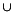Cup (union)
```\(ca ```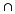Cap (intersection)
```\(sb ```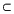Subset of
```\(sp ```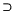Superset of
```\(ib ```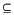Improper subset
```\(ip ```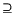Improper superset
```\(if ```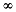Infinity
```\(pd ```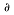Partial derivative
```\(gr ```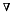Gradient
```\(no ``` ¬ Not
```\(is ```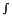Integral sign
```\(pt ```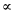Proportional to
```\(es ```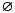Empty set
```\(mo ```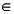Member of
```\(or ``` | Or

### 12.9.1 Greek Characters

Characters with equivalents as uppercase English letters are available on the standard fonts; otherwise, the characters in Table 12.6 exist only on the special font.

Table 12.6: Greek Characters
Input Char Char Name Input Char Char Name
```\(*a ```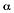alpha ```\(*A ``` A ALPHA
```\(*b ```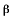beta ```\(*B ``` B BETA
```\(*g ```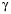gamma ```\(*G ```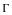GAMMA
```\(*d ```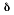delta ```\(*D ```DELTA
```\(*e ```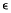epsilon ```\(*E ``` E EPSILON
```\(*z ```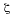zeta ```\(*Z ``` Z ZETA
```\(*y ```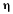eta ```\(*Y ``` H ETA
```\(*h ```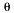theta ```\(*H ```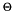THETA
```\(*i ```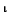iota ```\(*I ``` I IOTA
```\(*k ```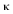kappa ```\(*K ``` K KAPPA
```\(*l ```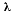lambda ```\(*L ```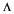LAMBDA
```\(*m ```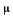mu ```\(*M ``` M MU
```\(*n ```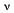nu ```\(*N ``` N NU
```\(*c ```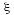xi ```\(*C ```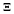XI
```\(*o ```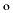omicron ```\(*O ``` O OMICRON
```\(*p ```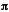pi ```\(*P ```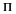PI
```\(*r ```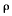rho ```\(*R ``` P RHO
```\(*s ```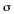sigma ```\(*S ```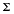SIGMA
```\(ts ```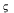terminal sigma
```\(*t ```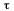tau ```\(*T ``` T TAU
```\(*u ```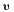upsilon ```\(*U ```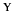UPSILON
```\(*f ```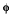phi ```\(*F ```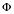PHI
```\(*x ```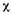chi ```\(*X ``` X CHI
```\(*q ```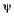psi ```\(*Q ```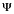PSI
```\(*w ```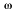omega ```\(*W ```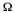OMEGA12.8 Predefined Registers13. mm Macros